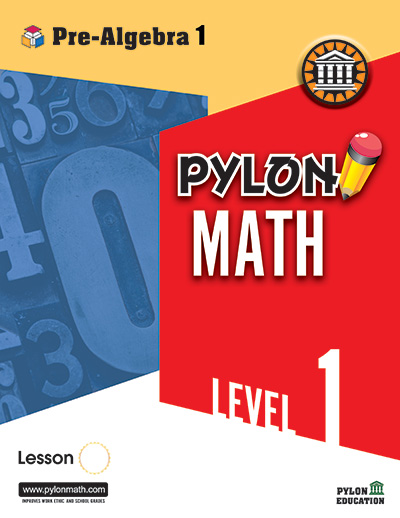# PreAlgebra 11. ### Variable Expressions

• Variable expressions
• Terms of variable expressions
• Evaluating variable expressions

### Powers

• Basic problems of powers
• Multiplication of exponential forms
• Multiplication of exponents
• Negative exponents
• Evaluating variable exponential forms
2. ### Order of Operations

• Basic operations
• Parentheses and exponents
• Exponents inside parentheses
• Parentheses, brackets, and braces
• Mixed problems

### Expanded Forms

• Expanded forms using positive exponents
• Expanded forms using negative exponents
3. ### Basic Properties and Equations

• Commutative, associative
• Distributive property
• Properties of zero and one

### Equations

• Inverse operations
• Making fractions for division
• Canceling denominators
• Mixed problems
• Two-step equations
• Combined operations
1. ### Prime and Composite Numbers

• Prime and composite numbers
• Prime factorization
• Prime factorization using exponents

### The Integers

• The sum of integers
• Subtracting integers
• Product of integers
• Quotient of integers
2. ### Capacity

• Converting capacity units
• Subtracting capacity units
• Metric system
• Changing units
3. ### Ratios

• Basic ratios
• Ratio of different units
• Unit rate
• Problem solving
• Equivalent ratios
• Proportions
• Scale drawing
• Problem solving
4. ### Percent Proportions

• Percents and hundredths
• Percents and decimals
• Changing fractions to percents
• Changing percents to fractions
• Percent proportions
5. ### Percent Equations

• Percent equations for parts
• Percent equations for rates
• Percent equations for bases
• Tax
• Discount